• I was wondering if there is an equivalent way to add a row to a Series or DataFrame with a MultiIndex as there is with a single index, i.e. using .ix or .loc?I thought the natural way would be somethi...I was wondering if there is an equivalent way to add a row to a Series or DataFrame with a MultiIndex as there is with a single index, i.e. using .ix or .loc?
I thought the natural way would be something like
but that raises a KeyError. I know I can use .append(), but I would find it much neater to use .ix[] or .loc[].
here an example:
>>> df = pd.DataFrame({'Time': [dt.datetime(2013,2,3,9,0,1), dt.datetime(2013,2,3,9,0,1)], 'hsec': [1,25], 'vals': [45,46]})
>>> df
Time hsec vals
0 2013-02-03 09:00:01 1 45
1 2013-02-03 09:00:01 25 46
[2 rows x 3 columns]
>>> df.set_index(['Time','hsec'],inplace=True)
>>> ind = pd.MultiIndex.from_tuples([(dt.datetime(2013,2,3,9,0,2),0)],names=['Time','hsec'])
>>> df.ix[ind] = 5
Traceback (most recent call last):
File "", line 1, in
df.ix[ind] = 5
File "C:\Program Files\Python27\lib\site-packages\pandas\core\indexing.py", line 96, in __setitem__
indexer = self._convert_to_indexer(key, is_setter=True)
File "C:\Program Files\Python27\lib\site-packages\pandas\core\indexing.py", line 967, in _convert_to_indexer
raise KeyError('%s not in index' % objarr[mask])
KeyError: "[(Timestamp('2013-02-03 09:00:02', tz=None), 0L)] not in index"
解决方案
You have to specify a tuple for the multi-indexing to work (AND you have to fully specify all axes, e.g. the : is necessary)
In : df.ix[(dt.datetime(2013,2,3,9,0,2),0),:] = 5
In : df
Out:
vals
Time hsec
2013-02-03 09:00:01 1 45
25 46
2013-02-03 09:00:02 0 5
Easier to reindex and/or concat/append a new dataframe though. Generally setting (with this kind of enlargement), only makes sense if you are doing it with a small number of values. As this makes a copy when you do this.

展开全文• 1. 查看名称, 重命名.name方法, 查看Series的名称. .rename()方法, 重命名import 数据类型为字符串, 原Series名称不变2. 查看Series头部数据, 尾部数据.head()方法, 查看Series头部(前几个)数据. .tail()方法, 查看...

1. 查看名称, 重命名
.name方法, 查看Series的名称. .rename()方法, 重命名

import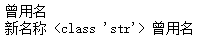数据类型为字符串, 原Series名称不变

2. 查看Series头部数据, 尾部数据

import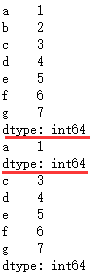3. Series数据索引 [超级重要]
3.0. 查看索引

import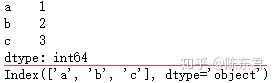3.1 标签索引

import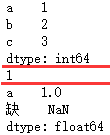如果index中不含索引的标签, 则返回NaN值

# 按属性,索引元素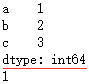3.2 下标索引
位置下标从0开始. 第一个元素下标索引为0

import pandas as pd
lst=[1,2,3]
s = pd.Series(lst,index=list("abc"))
print(s)
print(s[-2],type(s)) # 索引倒数第2个元素,并查看数据类型
print(s[[1,2]]) # 索引下标索引为1和2的元素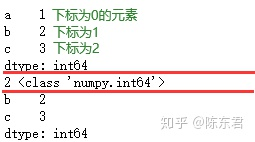3.3 切片索引

import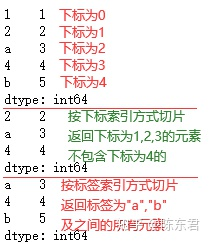切片索引与python中的list切片索引操作相同

import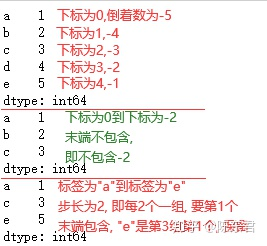3.4 布尔型索引
对Series进行判断, 可以生成布尔型的Series
布尔型索引, 保留生成的布尔型的Series中值为True的

import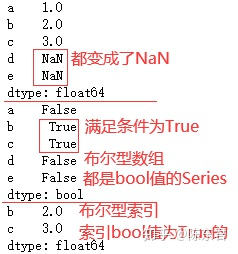.isnull()方法, 判断是否为空值

import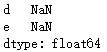.notnull()方法, 判断是否非空值, 用法同上
3.5. 重新索引

import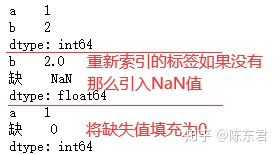4. Series排序
.sort_values()方法, 按值排序. .sort_index()方法, 按索引排序.

import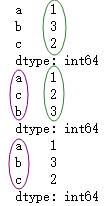4. Series对齐
Series之间的运算会按照index标签, 自动对齐, 与顺序无关.

import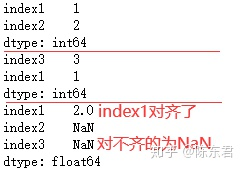5. 添加
5.1 标签索引添加

import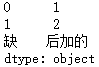5.2 .append(方法)添加

import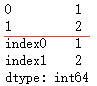6. 修改
标签索引修改

import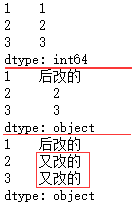7.删除
.drop()方法

import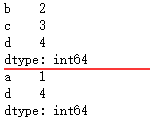详解
只索引单个元素时, 索引值必须包含在index中

import pandas as pd
lst=[1,2,3]
s = pd.Series(lst,index=list("abc"))
print(s)
print(s[["缺"]]) # index标签为"缺"的元素,组成的Series
print(s["缺"]) # index标签为"缺"的元素,由于不包含,所以报错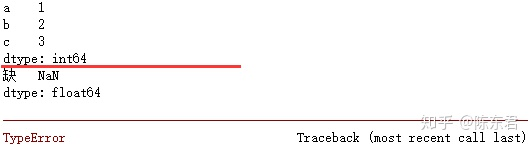得到Series, 由于不包含, 所以返回NaN值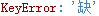部分报错内容, 大意就是index里没有*缺*这个key

纯数字的index, 不能用下标索引. python会认为是标签

import pandas as pd
lst=[1,2,3]
s = pd.Series(lst,index=[1,2,3])
print(s)
print(s) # 索引标签为1的元素,得到单个元素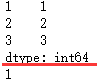展开全文• Series ： dataframe 中的每一行，或者每一列，就是一个 Series 理解 ： df > Series > value 图解 ： 2 ，一行Series if __name__ == '__main__': # 读数据 data = pd.read_csv("titanic_train.csv")...
1 ，Series 是什么 ：
dataframe ： pandas 读取 csv 文件后，得到 dfSeries ： dataframe 中的每一行，或者每一列，就是一个 Series理解 ： df > Series > value图解 ：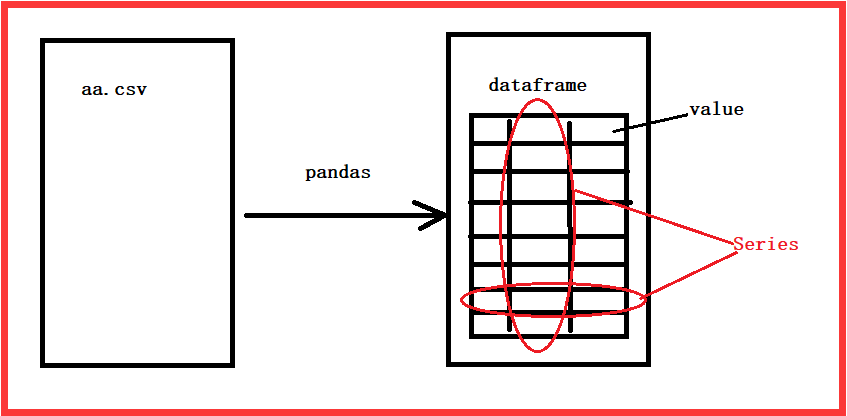2 ，一行 ： Series
if __name__ == '__main__':
# 读数据
# 是否成年列
res = data.loc
print(type(res))
结果 ：
<class 'pandas.core.series.Series'>

3 ，一列 ： Series
if __name__ == '__main__':
# 读数据
# 是否成年列
res = data["Age"]
print(type(res))
结果 ：
<class 'pandas.core.series.Series'>

4 ，Series 取值 ： res[0:1]
if __name__ == '__main__':
# 读数据
# 是否成年列
res = data["Age"]
print(res)
print(res[0:1])

5 ，Series 列出所有值 ：
列出 Series 中的所有值
if __name__ == '__main__':
# 读数据
# 是否成年列
ser = data.loc
print(ser)
print(ser.values)
结果 ：
PassengerId                          1
Survived                             0
Pclass                               3
Name           Braund, Mr. Owen Harris
Sex                               male
Age                                 22
SibSp                                1
Parch                                0
Ticket                       A/5 21171
Fare                              7.25
Cabin                              NaN
Embarked                             S
Name: 0, dtype: object
[1 0 3 'Braund, Mr. Owen Harris' 'male' 22.0 1 0 'A/5 21171' 7.25 nan 'S']

6 ，Series – ndarray ： Series 中的值的类型
if __name__ == '__main__':
# 读数据
# 是否成年列
ser = data.loc
nd = ser.values
print(type(ser))
print(type(nd))
结果 ：
<class 'pandas.core.series.Series'>
<class 'numpy.ndarray'>

7 ，Series 构成 ：
索引 ： index数据 ： ndarray
8 ，数据转换 ： list - ndarray - Series - dataframe
代码 ：
if __name__ == '__main__':
# 列表
li01 = ["a","b","c","d"]
li02 = ["张三","李四","王五","赵六"]
li03 = ["zs","ls","ww","zl"]
# ndarray
nd01 = np.array(li01)
nd02 = np.array(li02)
nd03 = np.array(li03)
# Series
ser01 = Series(nd02,index=nd01)
ser02 = Series(nd03,index=nd01)
# df ：同时给出列名
df01 = ser01.to_frame("name")
df02 = ser02.to_frame("en_name")
# 组装 ：
df01["en_name"] = df02
print(df01)

结果 ：
  name en_name
a   张三      zs
b   李四      ls
c   王五      ww
d   赵六      zl

9 ，自定义索引 ：
观察可知 ： 我们的数据的索引是 a,b,c,d 使我们自己定义的索引默认索引 ： 0,1,2,3,4…通过索引拿数据 ： r = df01.loc[“a”]
if __name__ == '__main__':
# 列表
li01 = ["a","b","c","d"]
li02 = ["张三","李四","王五","赵六"]
li03 = ["zs","ls","ww","zl"]
# ndarray
nd01 = np.array(li01)
nd02 = np.array(li02)
nd03 = np.array(li03)
# Series
ser01 = Series(nd02,index=nd01)
ser02 = Series(nd03,index=nd01)
# df ：同时给出列名
df01 = ser01.to_frame("name")
df02 = ser02.to_frame("en_name")
# 组装 ：
df01["en_name"] = df02
print(df01)
# 通过索引拿数据 ：
r = df01.loc["a"]
print(r)

结果 ：
name       张三
en_name    zs
Name: a, dtype: object

10 ，数据转换 ： dataframe - Series - ndarray - list
代码 ：
if __name__ == '__main__':
# df
# series
ser = df.loc
# ndarray
ndar = ser.values
li = ndar.tolist()

print(type(df))
print(type(ser))
print(type(ndar))
print(type(li))

结果 ：
<class 'pandas.core.frame.DataFrame'>
<class 'pandas.core.series.Series'>
<class 'numpy.ndarray'>
<class 'list'>

11 ，行列取值 ：
取一行 ：用索引
r = df01.loc["a"]

取一列 ：用列名
r02 = df01["name"]

展开全文python
• 记录学习路上的点滴，周，月，一年...总会有收获的，不当工作，只为兴趣。写在开始之前 本文和后续文章用到的数据是从 UCI公开数据网站 下载的 红酒数据集 。这个网站也是数据分析挖掘等领域入门的常用数据集...数据是可视化展示的实体，因此要会利用现成Python库进行数据读取和后续处理操作。
记录学习路上的点滴，一周，一月，一年...总会有收获的，不当工作，只为兴趣。写在开始之前
本文和后续文章用到的数据是从 UCI公开数据网站 下载的 红酒数据集 。这个网站也是数据分析挖掘等领域入门的常用数据集下载网站，数据可以说非常“干净”，适用于新手入门(说的就是和我一类的人，wahaha~)。
一般下载好的数据集，后缀名一般为.data，Pandas不能直接处理，怎么办？解决方案：

Excel如何导入外部数据-百度经验​jingyan.baidu.com另，想骂人= =||......Windows操作系统真的无语，经常遇到一些莫名其妙的问题，我今天就为了一个文件读取路径的Error，差点砸了电脑......为了避免这种尴尬，初学阶段，建议将数据和代码放在同一个文件夹下，尽可能避免莫名其妙的事情发生（linux和ios操作系统不用管...）。这样做，最大的好处在于，不会让你放弃Python。

Pandas数据读取
Pandas能够读取的数据类型为表格类型数据
这里以读取excel文件为例（用的最多的），其他基本相同。
原始Excel数据如下：（原本标签和数据是分开的，为了便于学习，我自己整合加了标签）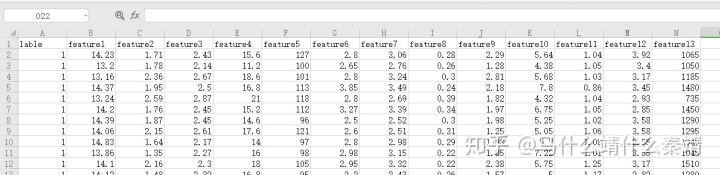import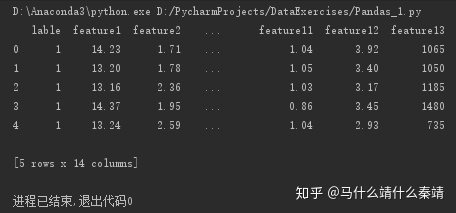这是一个三分类数据集，第1列是标签（把三种红酒用1、2、3进行了表示），第2至14列为特征属性（我们先不用管这些属性代表的实际意义）
下面是一些查看读入数据的基本操作：（非常实用，让你直观对数据有一个大体了解）
wine.shape # 查看数据的形状，返回(行数、列数)wine.columns # 查看列名列表（读入数据中有列名都是什么）wine.index # 查看索引列（多少行/条数据）wine.dtypes # 查看每列的数据类型

print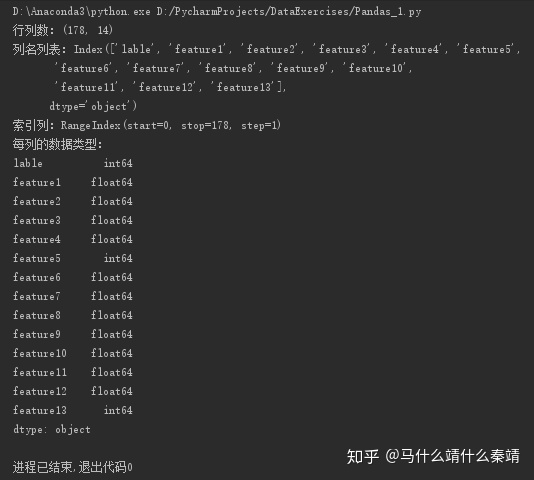Pandas的数据结构
Pandas的数据结构分为Series和DataFrame
Series是一种类似于一维数组的数据结构，它由一组数据（不同数据类型）以及一组与之相关的数据标签（即索引）组成DataFrame是一种表格型的数据结构。每列可以是不同的值类型（数值、字符串、布尔值等）；既有行索引index,也有列索引columns；可以被看做由Series组成的字典
分别讲解
一、Series
1、利用单一数据列表创建Series

s1_data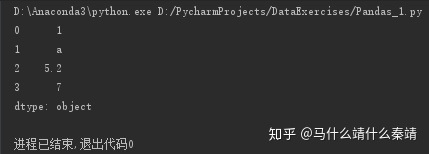由结果可以看到，左侧为索引（默认索引，是自动添加的），右侧为数据。
2、创建一个含有标签索引的Series

s2_data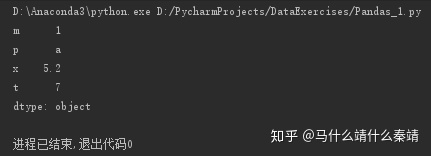3、使用Python字典创建Series

data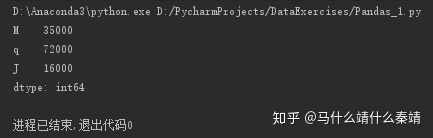Series类型数据结构可以使用 s1_data.index 获取索引，使用 s1_data.values 获取数据
4、Series依据索引查询数据
该方法非常类似于Python的字典dict
s3_data['q'] → 72000
type(s3_data['q']) → int64（这样查询到的是一个int64型的数值）
s3_data[['q'],['J']] → 72000 16000
type(s3_data[['q'],['J']]) → pandas.core.series.Series（这样查询到的仍然是一个Series数据结构）
二、DataFrame
1、利用多个字典序列创建DataFrame

data_2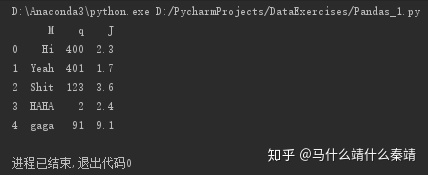利用前面所说的查看读入数据的基本操作，可以看看这个DataFrame大体是个什么样子，这里不再赘述。
3、在DataFrame中进行Series的查询操作
如果只查询一行、一列，返回的是pd.Series如果查询多行、多列，返回的是pd.DataFrame
记住上述两句话，不至于后面对数据进行操作时发生一些莫名其妙的错误！

print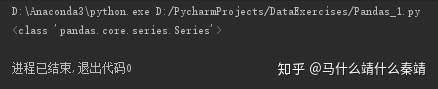可以看到是个Series类型

print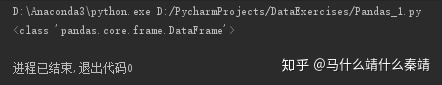可以看到是个DataFrame类型
同理，行查询，用df.loc[1:3]可以查询第一行到第三行的数据

print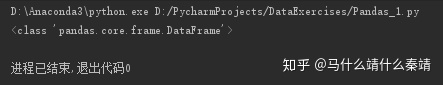多行为DataFrame类型，同理单行为Series类型

End

展开全文• 、介绍pandas 是基于NumPy的种工具，该工具是为了解决数据分析任务而创建的。Pandas 纳入了大量库和一些标准的数据模型，提供了高效地操作...2. pandas中提供了两种常见的数据类型：Series二维 DataFram...
• 二 pandas清洗数据一览数据面貌df获取查询数据末尾5pd.value_counts(Series)统计Series中不同元素出现的次数data各个年级出现的次数reset_index将serives转化为dataframedata=data.reset_index()duplicated 定位...
• 1、删除一行、一列 >>> df A B C D 0 1 3 3 4 1 5 6 7 8 2 1 1 1 1 3 2 3 2 3 #删除A列，不改变原来的data数据，返回删除后的新表data_2。axis为1表示删除列，0表示删除行。inplace为True表示直接对原...pandas dataframe 一列
• ## 往Series添加DataFrame

千次阅读 2018-08-09 05:28:09
Series添加DataFrame要注意的是Series的索引和DataFrame的列名是否匹配，当匹配时 s = pd.Series([1, 2]) df = pd.DataFrame({ 0: [10,10], 1: [10,10] }) print s print df print df + s 0 1 1 2 dtyp.....
• for循环将Series作为新插入DataFrame 导入包 from pandas import DataFrame import pandas as pd import numpy as np 创建df df = DataFrame(np.random.randn(4, 5), columns=['A', 'B', 'C', 'D', 'E']) print...python 索引
• 需求：写个汇总 分析：concat, merge, df.loc[xx] = [a,b,c] 都可以满足 也可像 list 一样用append 1.append(series) data = pd.DataFrame() series = pd.Series({"x":1,"y":2},name="a") data = data.append...
• 】●series相加我们知道两个字符串相加就会拼接成个新的字符串，那么pandas中dataframe的两个series相加是什么结果呢？如果这个dataframe的具体的某个数据的dtype是int和float类型，相加的效果就相当于两个数...
• import pandas as pd ...series = pd.Series({"x":1,"y":2},name="a") data = data.append(series) print(data) data = data.append(series) print(data) x y a 1.0 2.0 x y a 1.0 2.0 a 1.0 2.0
• 实例如下所示： from pandas import * from random import * df = DataFrame(columns=('lib', 'qty1', 'qty2'))#...以上这篇python实现在pandas.DataFrame添加一行就是小编分享给大家的全部内容了，希望能给大家一个参
• ## pandas 增加一行数据

万次阅读 2019-01-07 18:47:24
# 对于完整的自增索引，可以使用len(df)，在最后添加一行 for i in range(5): df.loc[len(df)] = [randint(-1,1) for n in range(3)] 这是一种方法，但是如果我们是往已有的DataFrame中添加数据，而已有的...
• 想给个原有的excel表格上实现添加新数据，但是由于刚学，不熟悉dataframe的特性，本来想按照写入json转csv的方式对数据进行添加，那就意味着要对原先表格的数据进行提取再series合并，最后再写入个新表格，...python pandas 数据分析
• #1楼 参考：https://stackoom.com/question/ixi9/向pandas-DataFrame添加一行 #2楼 You could use pandas.concat() or DataFrame.append() . 您可以使用pandas.concat()或DataFrame.append() 。 For details and ...python pandas dataframe append
• Series种类似于维数组的对象，是由组数据(各种NumPy数据类型)以及组与之相关的数据标签(即索引)组成。仅由组数据也可产生简单的Series对象。注意：Series中的索引值是可以重复的。 DataFrame：个表格...
• 1.创建多层索引 (1) 隐式构造 最常见的方法是给DataFrame构造函数的index参数传递两个或更多的数组 -- Series也可以创建多层索引 import numpy as np import matplotlib.pyplot as plt import pandas as ...Pandas
• 1.可以把Series看成一个定长的有序字典，向Series增加一行：相当于给字典增加一组键值对。 2.可以通过shape，size，index,values等得到series的属性 shape：形状 size：元素的个数 index：获取index的值，返回的是...python pandas
• 在生成了Bi后，想把Bi的每一行都传给A，不断有B1 B2 B3...，在A上增加行 本来以为可以直接通过增加行的代码，比如 A.iloc[0,:] = B.iloc[0,:] 但就是跑不通 反反复复尝试最后的版本变为： fo...
• Series格式和ndarray格式中，提取特定的列数据读取数据Series中提取某列数据ndarray中提取某列数据数据集的图表展示 本文使用的数据集是鸢尾花，代码写在jupy notebook中。 数据集内包含 3 类共 150 条记录，...数据分析 DataFrame ndarray
• 代码示例： from pandas import DataFrame,Series city={ 'Province': ['TianJin', 'Beijing', 'ShangHai', 'ChongQing','ShenZhen'], 'pop': [1.3, 2.5, 1.9, 0.7,2.1], ...#DataFrime的一行或者一列都是Seriespandas
• 、Pandas概述 1.1 简介 Pandas是Python生态中非常重要的数据分析包，它是个开源的库，...Series（类似于维数组） DataFrame（类似于二维数组） Panel（类似于三维数组）。由于Panel并不常用，因此，新版本的PaPython pandas dataframe 数据类型
• >df = DataFrame(columns=('lib', 'qty1', 'qty2')) for i in range(5): df.loc[i] = [randint(-1,1) for n in range(3)] >print(df) lib qty1 qty2 0 0 0 -1 1 -1 -1 1 2 1 -1 1 3 0 0 0 4 1 -1 -1 ...
• 23_Pandas.DataFrame,Series中提取・删除重复 从pandas.DataFrame和pandas.Series提取包含重复元素的方法是duplicated(),删除包含重复元素的方法是drop_duplicates（）以将其删除。 以下面的数据为例。添加...python 机器学习
• 本文所用的teechart控件为teechart8.ocx 假设与teechart控件绑定的控件变量为m_chart m_chart.AddSeries(0);...CSeries datax=m_chart.Series(0); //这里的0是指插入的series序列 datax.put_Color(RGB(255,...
• pandas:基于numpy，生成两种对象，种是series,种是dataframe。 series: 维数组类似array,series=索引+数据。区别是Series能保存不同种数据类型，字符串、boolean值、数字等，而numpy只能存储同类型数...python
• 从DataFrame中取出一行或者一列 1.1、 使用pd.Series() 第二种方法主要在DataFrame的讲解中介绍，这里主要讲解使用前者pd.Series()，里面的参数可以是普通的列表，也可以是字典、其他的ndarray或者标量值。 使用......